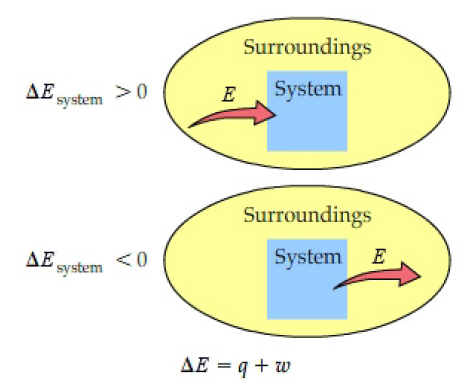# Problem: An ideal gaseous reaction (which is a hypothetical gaseous reaction that conforms to the laws governing gas behavior) occurs at a constant pressure of 50.0 atm and releases 72.5 kJ of heat. Before the reaction, the volume of the system was 8.80 L. After the reaction, the volume of the system was 2.00 L. Calculate the total internal energy change, ΔE, in kilojoules.Express your answer with the appropriate units.Energy cannot be created nor destroyed, but it can be transferred between a system and its surroundings. The change in internal energy, ΔE, is positive if the system absorbs energy, and it is negative if the system releases energy. The total change in internal energy is the sum of the heat, q, and work, w: ΔE = q + w.

###### FREE Expert Solution
93% (396 ratings)View Complete Written Solution
###### Problem Details

An ideal gaseous reaction (which is a hypothetical gaseous reaction that conforms to the laws governing gas behavior) occurs at a constant pressure of 50.0 atm and releases 72.5 kJ of heat. Before the reaction, the volume of the system was 8.80 L. After the reaction, the volume of the system was 2.00 L. Calculate the total internal energy change, ΔE, in kilojoules.